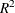# The SYSLIN Procedure

### Two-Stage Least Squares Estimation

In the supply and demand model, P is an endogenous variable, and consequently the OLS estimates are biased. The following example estimates this model using two-stage least squares.

```proc syslin data=in 2sls;
endogenous  p;
instruments y u s;
demand: model q = p y s;
supply: model q = p u;
run;
```

The 2SLS option in the PROC SYSLIN statement specifies the two-stage least squares method. The ENDOGENOUS statement specifies that P is an endogenous regressor for which first-stage predicted values are substituted. You need to declare an endogenous variable in the ENDOGENOUS statement only if it is used as a regressor; thus although Q is endogenous in this model, it is not necessary to list it in the ENDOGENOUS statement.

Usually, all predetermined variables that appear in the system are used as instruments. The INSTRUMENTS statement specifies that the exogenous variables Y, U, and S are used as instruments for the first-stage regression to predict P.

The 2SLS results are shown in Figure 29.3 and Figure 29.4. The first-stage regressions are not shown. To see the first-stage regression results, use the FIRST option in the PROC SYSLIN statement.

Figure 29.3: 2SLS Results for Demand Equation

The SYSLIN Procedure
Two-Stage Least Squares Estimation

Model DEMAND q Quantity

Analysis of Variance
Source DF Sum of Squares Mean Square F Value Pr > F
Model 3 9.670892 3.223631 115.58 <.0001
Error 56 1.561956 0.027892
Corrected Total 59 10.03724

 Root MSE R-Square 0.16701 0.86095 1.30095 0.85350 12.8374

Parameter Estimates
Variable DF Parameter
Estimate
Standard
Error
t Value Pr > |t| Variable
Label
Intercept 1 1.901048 1.171231 1.62 0.1102 Intercept
p 1 -1.11519 0.607395 -1.84 0.0717 Price
y 1 0.419546 0.117955 3.56 0.0008 Income
s 1 0.331475 0.088472 3.75 0.0004 Price of Substitutes

Figure 29.4: 2SLS Results for Supply Equation

The SYSLIN Procedure
Two-Stage Least Squares Estimation

Model SUPPLY q Quantity

Analysis of Variance
Source DF Sum of Squares Mean Square F Value Pr > F
Model 2 9.646109 4.823054 253.96 <.0001
Error 57 1.082503 0.018991
Corrected Total 59 10.03724

 Root MSE R-Square 0.13781 0.89910 1.30095 0.89556 10.5929

Parameter Estimates
Variable DF Parameter
Estimate
Standard
Error
t Value Pr > |t| Variable
Label
Intercept 1 -0.51878 0.490999 -1.06 0.2952 Intercept
p 1 1.333080 0.059271 22.49 <.0001 Price
u 1 -1.14623 0.243491 -4.71 <.0001 Unit Cost

The 2SLS output is similar in form to the OLS output. However, the 2SLS results are based on predicted values for the endogenous regressors from the first stage instrumental regressions. This makes the analysis-of-variance table and thestatistics difficult to interpret. See the sections ANOVA Table for Instrumental Variables Methods and The R-Square Statistics for details.

Note that, unlike the OLS results, the 2SLS estimate for the P coefficient in the demand equation (–1.115) is negative.